# Math Practice Topic: Proportions 2

 Description: This topic applies proportions to solve problems with shapes. Adaptive Learning Progression: Uses various shapes. Start using MathScore for free

## Sample Levels (out of 4)

Find the value of 'n'.

 1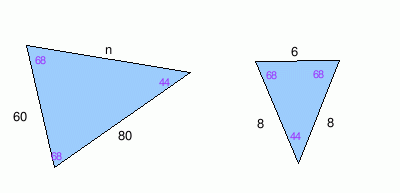n = 2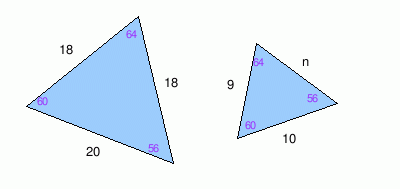n =

Find the value of 'n'.

 1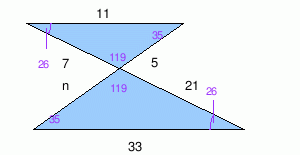n = 2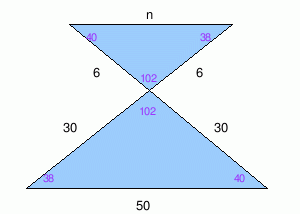n =

Find the value of 'n'.

 1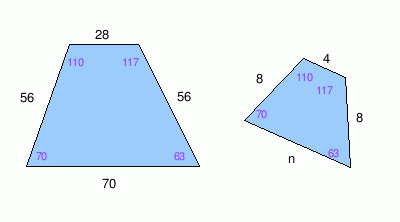n = 2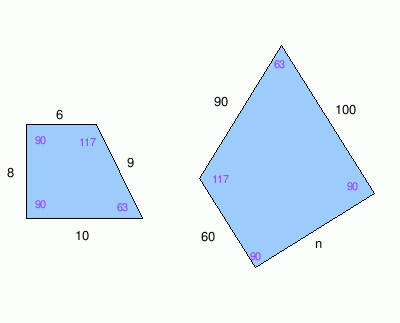n =

Find the value of 'n'.

 1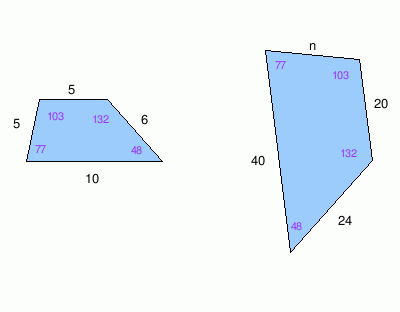n = 2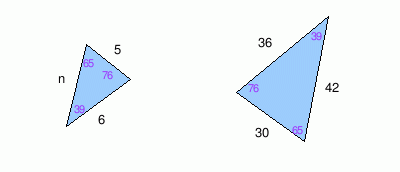n =